# ML Aggarwal Class 6 Solutions for ICSE Maths Chapter 10 Basic Geometrical Concept Ex 10.3

## ML Aggarwal Class 6 Solutions for ICSE Maths Chapter 10 Basic Geometrical Concept Ex 10.3

Question 1.
Draw rough diagrams to illustrate the following:
(i) open simple curve
(ii) closed simple curve
(iii) open curve that is not simple
(iv) closed curve that is not simple.
Solution: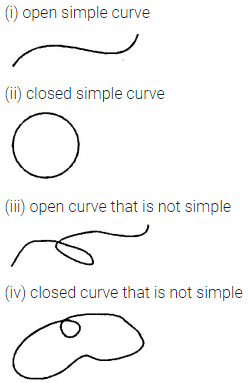Question 2.
Consider the given figure and answer the following questions:
(i) Is it a curve?
(ii) Is it a closed curve?
(iii) Is it a polygon?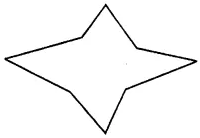Solution:Question 3.
Draw a rough sketch of a triangle ABC. Mark a point P in its interior and a point Q in its exterior. Is the point A in its exterior or in its interior?
Solution: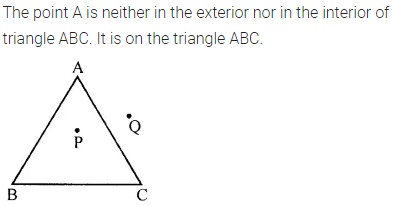Question 4.
Draw a rough sketch of a quadrilateral PQRS. Draw its diagonals. Name them.
Solution:Question 5.
In context of the given figure:
(i) Is it a simple closed curve?
(iii) Draw its diagonals and name them.
(iv) State which diagonal lies in the interior and which diagonal lies in the exterior of the quadrilateral.Solution: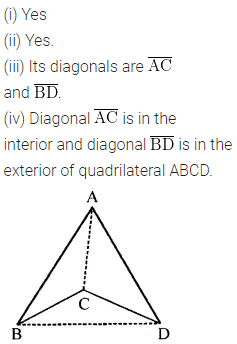Question 6.
Draw a rough sketch of a quadrilateral KLMN. State,
(i) two pairs of opposite sides
(ii) two pairs of opposite angles
(iii) two pairs of adjacent sides
(iv) two pairs of adjacent angles.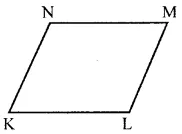Solution: